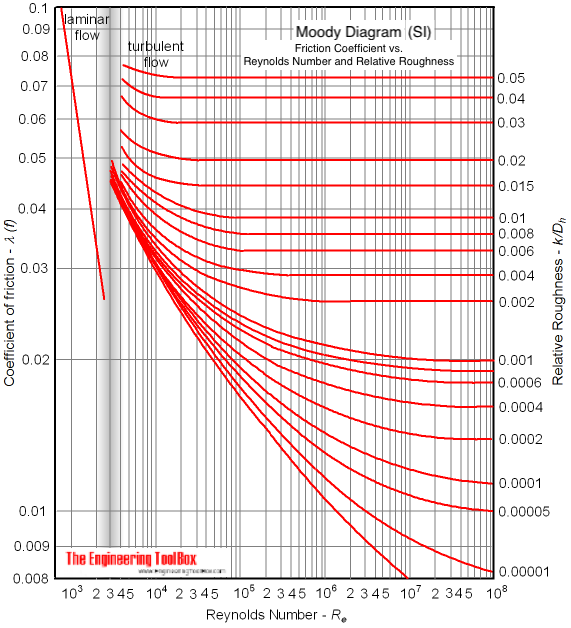Engineering ToolBox - Resources, Tools and Basic Information for Engineering and Design of Technical Applications!

# Laminar Flow - Friction Coefficients

## Calculate friction coefficients for laminar fluid flow.

The friction coefficient - or factor - of a fluid flow at laminar conditions can be calculated as

λ = 64 / Re

= 64 μ / (d hu ρ)

= 64 ν / (d hu)                          (1)

where

λ = friction coefficient (non-dimensional)

Re = Reynolds Number (non-dimensional)

μ = absolute (dynamic) viscosity (Ns/m 2 , lb m/s ft)

d h= hydraulic diameter (mm, ft)

u = mean velocity in flow (m/s, ft/s)

ρ = density of fluid (kg/m 3 , lb m/ft 3 )

ν = μ / ρ = kinematic viscosity (m 2 /s, ft 2 /s)

Equation (1) is only valid at laminar conditions where Reynolds Number is less than 2300 . For turbulent conditions where Reynolds Number exceeds 4000 the Colebrook equation should be used to calculate the friction coefficient.

In practice laminar flow is only actual for viscous fluids - like crude oil, fuel oil and other oils.

The friction coefficient for laminar flow indicated in the Moody diagram (SI based):## Related Topics

• ### Fluid Mechanics

The study of fluids - liquids and gases. Involving velocity, pressure, density and temperature as functions of space and time.

## Related Documents

• ### Colebrook Equation

Friction loss coefficients in pipes, tubes and ducts.
• ### Darcy-Weisbach Equation - Major Pressure and Head Loss due to Friction

The Darcy-Weisbach equation can be used to calculate the major pressure and head loss due to friction in ducts, pipes or tubes.
• ### Reynolds Number

Introduction and definition of the dimensionless Reynolds Number - online calculators.

## Search

Search is the most efficient way to navigate the Engineering ToolBox.

## Engineering ToolBox - SketchUp Extension - Online 3D modeling!

Add standard and customized parametric components - like flange beams, lumbers, piping, stairs and more - to your Sketchup model with the Engineering ToolBox - SketchUp Extension - enabled for use with older versions of the amazing SketchUp Make and the newer "up to date" SketchUp Pro . Add the Engineering ToolBox extension to your SketchUp Make/Pro from the Extension Warehouse !

We don't collect information from our users. More about

## Citation

• The Engineering ToolBox (2008). Laminar Flow - Friction Coefficients. [online] Available at: https://www.engineeringtoolbox.com/laminar-friction-coefficient-d_1032.html [Accessed Day Month Year].

Modify the access date according your visit.

12.8.9